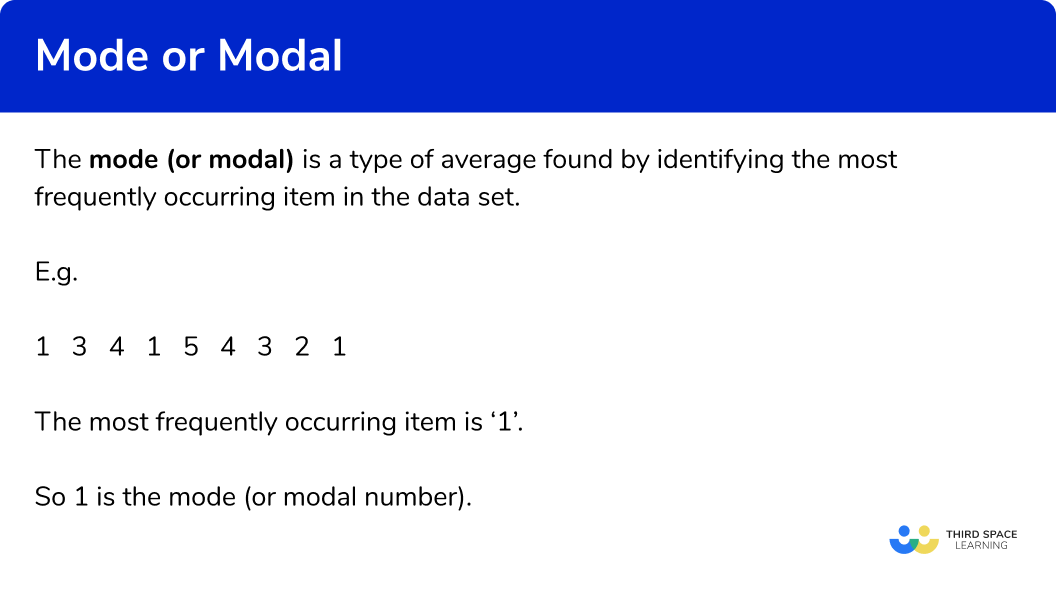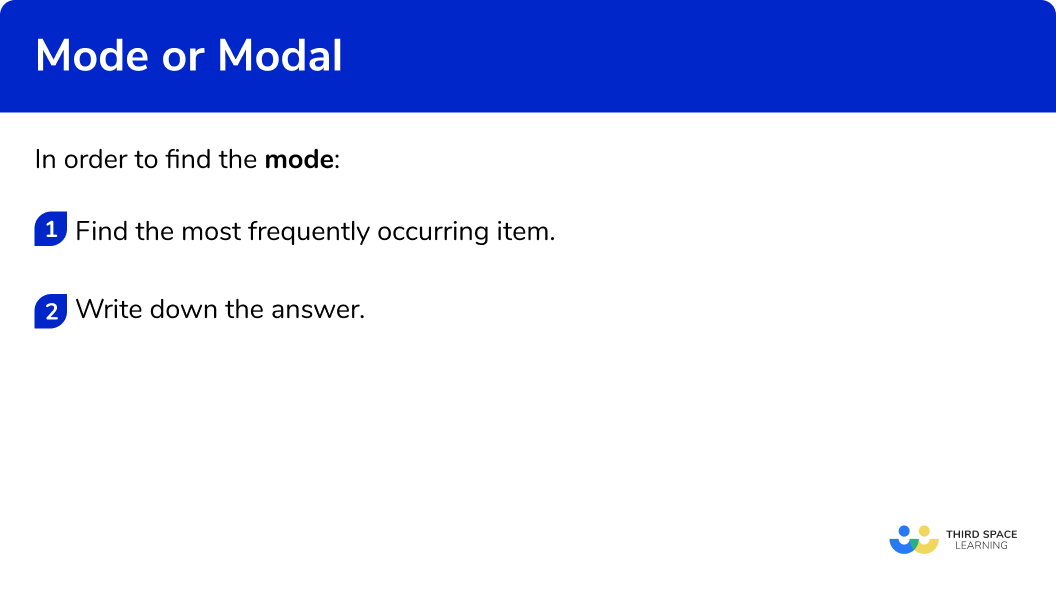# Mode Or Modal

Here we will learn about the mode or modal in maths, including what is and how to find it.

There are also mode worksheets based on Edexcel, AQA and OCR exam questions, along with further guidance on where to go next if you’re still stuck.

## What is the mode or modal?

The mode (or modal) is a type of average found by identifying the most frequently occurring item in the data set.

E.g. Find the mode

The most frequently occurring item is ‘1’ .

So 1 is the mode (or modal number).

The mode is special as it is the only type of average which can be used with categorical data. Categorical data uses words rather than numbers.

E.g. Find the mode

The most frequently occurring item is ‘blue’ – so ‘blue’ is the mode.

It is also the only average where there can be two different values.  When this happens we say the data is bimodal.

E.g. Find the mode

The most frequently occurring item is ‘1’ and ‘3’ – so 1 and 3 are the modes (or modal number). This data is bimodal as it has 2 modes.

The mode or modal value is a measure of central tendency because it describes a set of numbers by identifying a central position within the data.

### What is the mode or modal?## How to find the mode

In order to find the mode:

1. Find the most frequently occurring item.

### How to find the mode## Related lessons on mean, median, mode

Mode or modal is part of our series of lessons to support revision on mean, median, mode. You may find it helpful to start with the main mean, median, mode lesson for a summary of what to expect, or use the step by step guides below for further detail on individual topics. Other lessons in this series include:

## Mode maths or modal maths examples

### Example 1: categorical data

Find the mode of this data set:

1. Find the most frequently occurring item.

You will need to count the number of times the different words occur. The most common item is ‘red’. The number of times ‘red’ occurs is 3 times.

The mode is red

### Example 2: categorical data

Find the mode of this data set:

You will need to count the number of times the different words occur. The most common item is ‘apple’. The number of times ‘apple’ occurs is 4 times.

The mode is apple

### Example 3: numerical data

Find the mode of this list of number:

You will need to count the number of times the different values occur. The most common item is ‘4’ . The number of times ‘4’ occurs is 3 times.

The mode (or modal value) is 4

### Example 4: numerical data

Find the mode of this list of number:

You will need to count the number of times the different values occur. The most common item is ‘25’ . The number of times ‘25’ occurs is 4 times.

The mode (or modal value) is 25

### Example 5: bimodal

Find the mode of this list of number:

You will need to count the number of times the different values occur. The most common items are ‘3’ and ‘5’ . They both occur 2 times and the other values only occur once.

The mode (or modal value) is 3 and 5

### Example 6: bimodal

Find the mode of this list of number:

You will need to count the number of times the different values occur. The most common items are ‘1’ and ‘3’ . They both occur 3 times and the other values only occur once.

The mode (or modal value) is 1 and 3

### Common misconceptions

• Check which average you are being asked for

Check if you have been asked for the median, mode or mean average.

• No mode?

A data set can have 1 mode or 2 modes (bimodal). But it is possible that a data set has no mode at all.

• Item from the data set

The mode will be the item – NOT the frequency.

E.g.

Find the mode:

There are 3 A s, 2 B s and 6 C s.  The item which occurs the most often is ‘C’ . So the mode is ‘C’ . As ‘C’ occurs 6 times the frequency of C is 6 .

### Practice mode maths or modal maths questions

1. Find the mode:redblueyellowgreenThe most common item is ‘yellow’. The number of times ‘yellow’ occurs is 2 times. The other colours only occur once.

2. Find the mode:MediumLargeSmallThere is no modeThe most common item is ‘small’. The number of times ‘small’ occurs is 3 times. ‘Medium’ occurs twice and ‘large’ once.

3. Find the mode:2341The most common item is ‘1’ . The number of times ‘1’ occurs is 2 times. The other numbers only occur once.

4. Find the mode of this set of data:622

and

6There is no mode.The most common item is ‘2’ . The number of times ‘2’ occurs is 3 times. The number of times ‘6’ occurs is 2 times, and the other numbers only occur once.

5. Find the mode of this set of numbers:12

and

14131214The most common items are 12 and 14 . They both occur twice. This data has two modes. It is bimodal.

6. Find the mode:10203010

and

30The most common items are 10 and 30 . They both occur 3 times. This data has two modes. It is bimodal.

### Mode maths or modal maths GCSE questions

1. Here is a list of numbers:Write down the mode.

(1 mark)

17

(1)

2. Here is a list of numbers:Write down the mode.

(1 mark)

25

(1)

3. Theo did a survey of the colour of the cars passing his house. The cars were only red, black, silver or blue.
The chart shows the number of red cars, the number of black cars and the number of silver cars.The total number of cars is 25 .

(a) Work out the number of blue cars.
(b) Write down the mode.

(3 marks)

(a)
25-(7+5+9)
For subtracting the numbers of red, black and silver cars from the total number of cars

(1)

=4

(1)

(b)
Silver

(1)

## Learning checklist

You have now learned how to:

• Find the mode for categorical data
• Find the mode (or modal number) for numerical data

## Still stuck?

Prepare your KS4 students for maths GCSEs success with Third Space Learning. Weekly online one to one GCSE maths revision lessons delivered by expert maths tutors.

Find out more about our GCSE maths tuition programme.